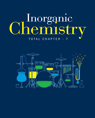×#### Thank you for registering.

One of our academic counsellors will contact you within 1 working day.

Click to Chat

1800-1023-196

+91-120-4616500

CART 0

• 0

MY CART (5)

Use Coupon: CART20 and get 20% off on all online Study Material

ITEM
DETAILS
MRP
DISCOUNT
FINAL PRICE
Total Price: Rs.

There are no items in this cart.
Continue Shopping
```
If in a question in equilibrium chapter,we have asked to find out the equilibrium constant for a reaction like compound A gives rise to two compounds 2B+C given that number of moles of compound A is 4 moles but at equilibrium only 50% of compound A is dissociated into 2B and C. Then how will we find equilibrium constant (Kc).
If in a question in equilibrium chapter,we have asked to find out the equilibrium constant for a reaction like compound A gives rise to two compounds 2B+C given that number of moles of compound A is 4 moles but at equilibrium only 50% of compound A is dissociated into 2B and C. Then how will we find equilibrium constant (Kc).

```
3 years ago

```							A--->2B+C   Then equilibrium constant Kc is given as   {[B]^2 [C]/[A]}Now according to stichiometry if 1 mole of A is consumed them 2 mole of B is formed and 1 mole of C is Therefore now 50% of A is consumed i.e 2 moles thus 4 mole of B & 2 mole of C is formed therefore Kc={(4)^2×2/2 }=Here (4)^2 refers square of 4 =16&[B] is concentration of B at equilibrium Thank u
```
3 years ago
Think You Can Provide A Better Answer ?

## Other Related Questions on Inorganic Chemistry

View all Questions »### Course Features

• 731 Video Lectures
• Revision Notes
• Previous Year Papers
• Mind Map
• Study Planner
• NCERT Solutions
• Discussion Forum
• Test paper with Video Solution### Course Features

• 54 Video Lectures
• Revision Notes
• Test paper with Video Solution
• Mind Map
• Study Planner
• NCERT Solutions
• Discussion Forum
• Previous Year Exam Questions